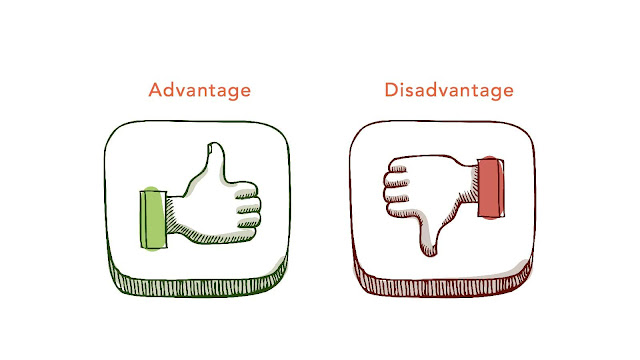# HSSlive: Plus One & Plus Two Notes & Solutions for Kerala State Board

## Monday, March 13, 2023Advantages And Disadvantages Of 2D Rectangular Matrix In Java

## What is 2D Rectangular Matrix In Java?

Java, a 2D rectangular matrix is a two-dimensional array with rows and columns that are organized in a rectangular shape.

To create a 2D rectangular matrix in Java, you need to declare a two-dimensional array and specify the number of rows and columns. For example, to create a 2D matrix with 3 rows and 4 columns, you can use the following code:

`int[][] matrix = new int; `

This creates a 2D rectangular matrix with 3 rows and 4 columns, where each element in the matrix is initialized to the default value of 0. You can access individual elements in the matrix using their row and column indices. For example, to access the element in the second row and third column of the matrix, you can use the following code:

`int element = matrix; // row index 1 corresponds to the second row, column index 2 corresponds to the third column `

## Advantages of 2D Rectangular Matrix In Java

There are several advantages to using a 2D rectangular matrix in Java, including:

1. Easy to understand: A 2D rectangular matrix is a common data structure used in many computer science applications. It is easy to understand, and programmers can quickly visualize the data they are working with.

2. Efficient storage: In Java, a 2D rectangular matrix is implemented as an array of arrays. This means that the data is stored in a contiguous block of memory, making it efficient to access and manipulate.

3. Easy to access elements: Elements in a 2D rectangular matrix can be accessed using row and column indices, which are simple to understand and easy to work with.

4. Flexible: A 2D rectangular matrix can be used to represent many different types of data, such as images, tables, graphs, and more. It can also be resized dynamically, allowing for greater flexibility in programming.

5. Efficient algorithms: Many algorithms in computer science are designed to work with 2D rectangular matrices, such as matrix multiplication, image processing, and more. By using this data structure, programmers can take advantage of these efficient algorithms and write faster, more optimized code.

Overall, the 2D rectangular matrix is a powerful and versatile data structure in Java that provides many benefits to programmers.

## Disadvantages of 2D Rectangular Matrix In Java

While there are several advantages to using a 2D rectangular matrix in Java, there are also some potential disadvantages that programmers should be aware of, including:

1. Fixed size: In Java, the size of an array (including a 2D rectangular matrix) must be specified when it is created. This means that once the array is created, its size cannot be changed. This can be a disadvantage if you need to dynamically add or remove rows or columns from the matrix.

2. Uneven data: A 2D rectangular matrix assumes that all rows have the same number of columns. If your data does not fit this pattern, you may need to use a more complex data structure or pad the data with dummy values, which can be inefficient.

3. Limited functionality: While a 2D rectangular matrix is a useful data structure for many applications, it has some limitations. For example, it may not be the best choice for storing and manipulating complex data structures like graphs, trees, or networks.

4. Memory management: Because a 2D rectangular matrix is implemented as an array of arrays, it can be more challenging to manage memory efficiently. In some cases, you may need to manually allocate and deallocate memory to avoid memory leaks or performance issues.

5. Indexing complexity: While indexing elements in a 2D rectangular matrix is generally straightforward, it can become more complex when working with larger or more complex data sets. This can make it challenging to write efficient code that operates on the matrix quickly and accurately.

Overall, while a 2D rectangular matrix is a powerful and versatile data structure in Java, it may not be the best choice for all applications. Programmers should carefully consider the potential disadvantages and weigh them against the benefits before deciding to use a 2D rectangular matrix.

Share: